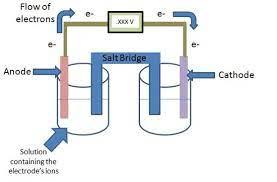## How to Calculate and Solve for Electrochemical Cell Potential | Corrosion

The electrochemical cell potential is illustrated by the image below.To compute for electrochemical cell potential, two essential parameters are needed and these parameters are Positive Cell Potential (V2°) and Negative Cell Potential (V1°).

The formula for calculating electrochemical cell potential:

ΔV° = V2° – V1°

Where:

ΔV° = Electrochemical Cell Potential
V2° = Positive Cell Potential
V1° = Negative Cell Potential

Given an example;
Find the electrochemical cell potential when the positive cell potential is 14 and the negative cell potential is 7.

This implies that;

V2° = Positive Cell Potential = 14
V1° = Negative Cell Potential = 7

ΔV° = V2° – V1°
ΔV° = 14 – 7
ΔV° = 7

Therefore, the elecreochemical cell potential is 7 V.

Calculating the Positive Cell Potential when the Electrochemical Cell Potential and the Negative Cell Potential is Given.

V2° = ΔV° + V1°

Where:

V2° = Positive Cell Potential
ΔV° = Electrochemical Cell Potential
V1° = Negative Cell Potential

Let’s solve an example;
Find the positive cell potential when the electrochemical cell potential is 20 and the negative cell potential is 14.

This implies that;

ΔV° = Electrochemical Cell Potential = 20
V1° = Negative Cell Potential = 14

V2° = ΔV° + V1°
V2° = 20 + 14
V2° = 34

Therefore, the positive cell potential is 34 V.